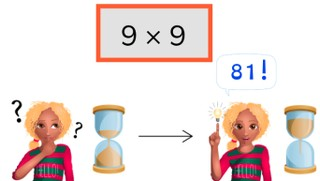Automaticity 9 times table

# Automaticity 9 times table

Students learn to memorize the 9 times table.

No account needed.8,000 schools use Gynzy92,000 teachers use Gynzy1,600,000 students use Gynzy

## General

The students memorize the 9 times table.

## Standards

CCSS.Math.Content.3.OA.C.7

## Learning objective

Students will be able to memorize the 9 times table and quickly give its products.

## Introduction

Drag the rabbit through the maze to the carrot. The rabbit can only take jumps of 9 (0, 9, 18, 27, etc.). He can only jump left or right and up or down. He cannot move diagonally.

## Instruction

First have the students solve problems from the 9 times table in 5, 4, and 3 seconds. Then show the pile of cards. Explain that you are going to start the timer and drag the cover away to show the first problem. Have the students solve the problem quickly and correctly, then drag the card away to show the next problem. Try to go through all the cards within the time given by the timer.

Check whether the students have memorized the 9 times table with the following question:
- Why is it important to memorize the 9 times table?

## Quiz

The students test their memorization of the 9 times table by completing 3 timed exercises.

## Closing

Discuss once again the importance of memorizing the 9 times table. Finally, ask the students how fast they think they can solve five multiplication problems from the 9 times table. Set the timer for that time, then spin the wheel 5 times and have the students solve the problems.

## Teaching tips

Give students that have difficulty memorizing the 9 times table a printed copy of the 9 times table. Have them practice in pairs with a timer, answering problems in shorter and shorter amounts of time.

## Instruction materials

You may print out a copy of the 9 times table.

### The online teaching platform for interactive whiteboards and displays in schools

• Save time building lessons

• Manage the classroom more efficiently

• Increase student engagement